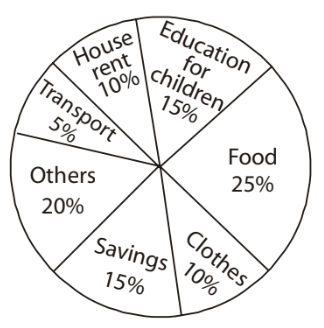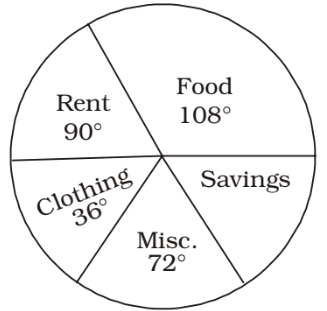## Introduction to Data Interpretation

#### Data Interpretation

Direction: Study the pie-chart and table given below and answer the questions.1. The percentage of the number of male employees working in Marketing department to the total number of employees in Marketing department is

1. Number of employees in Marketing department

 = 800 × 24 = 192 100

∴ Required percentage
 = 165 × 100 = 86 192

##### Correct Option: B

Number of employees in Marketing department

 = 800 × 24 = 192 100

∴ Required percentage
 = 165 × 100 = 86 192

1. The respective ratio between the number of females working in HR department to the total number of employees working in the HR department is

1. Number of employees in HR department

 = 800 × 5 = 40 100

##### Correct Option: A

Number of employees in HR department

 = 800 × 5 = 40 100

1. The pie - chart gives the expenditure (in percentage) on various items and savings of a family during a month. Monthly savings of the family is 3, 000. On which item is the expenditure maximum and how much is it?1. Expenditure of food = 25%
Savings = 15%
∵ 15% ≡ \$ 3000

 ∴ 25% ≡ 3000 × 25 = \$ 5000 15

##### Correct Option: D

Expenditure of food = 25%
Savings = 15%
∵ 15% ≡ \$ 3000

 ∴ 25% ≡ 3000 × 25 = \$ 5000 15

1. The following pie - chart shows the monthly expenditure of a family on food, clothing, rent, miscellaneous expenses and savings. What is the central angle for savings?1. Food + Rent + Clothing + Miscellaneous
⇒ 108 ° + 90° + 36° + 72° = 306°
∴ Savings ⇒ 360° – 306° = 54°.

##### Correct Option: A

Food + Rent + Clothing + Miscellaneous
⇒ 108 ° + 90° + 36° + 72° = 306°
∴ Savings ⇒ 360° – 306° = 54°.

Direction: The pie chart given below represents the modes of transport for 1400 officers of the Staff Selection Commission, Kolkata.
Study the chart and answer the following questions.1. The number of officers who go to office by car is

1. Number of car users

 ⇒ 1400 × 21 = 294 100

##### Correct Option: D

Number of car users

 ⇒ 1400 × 21 = 294 100